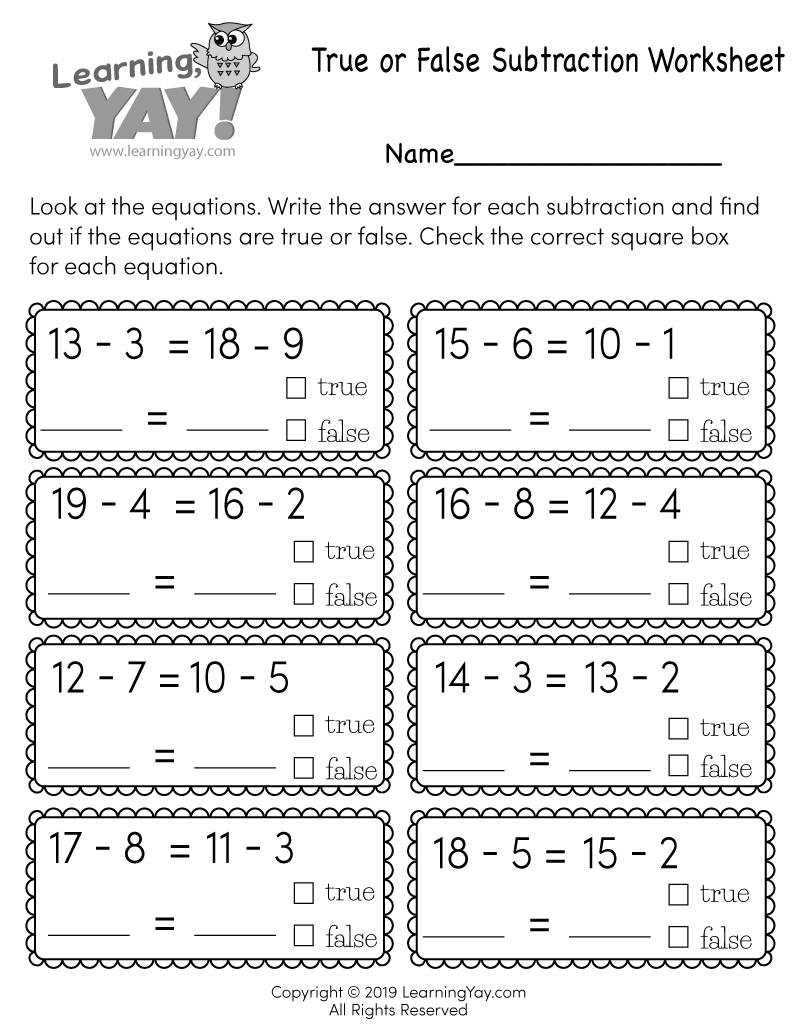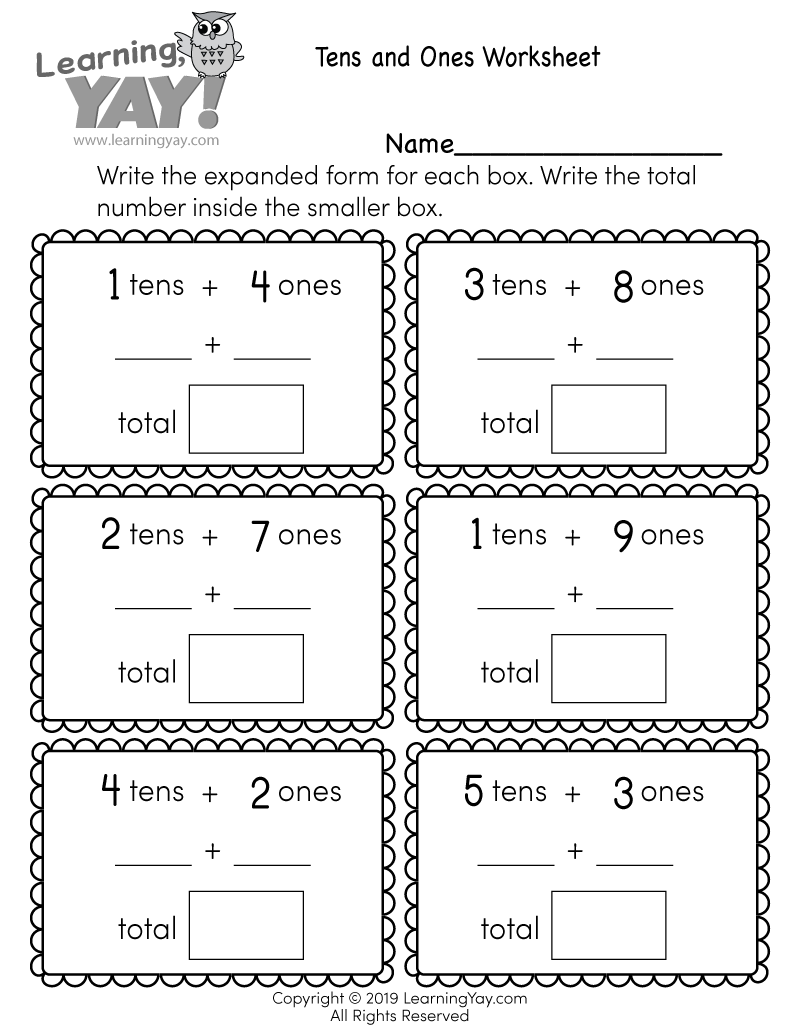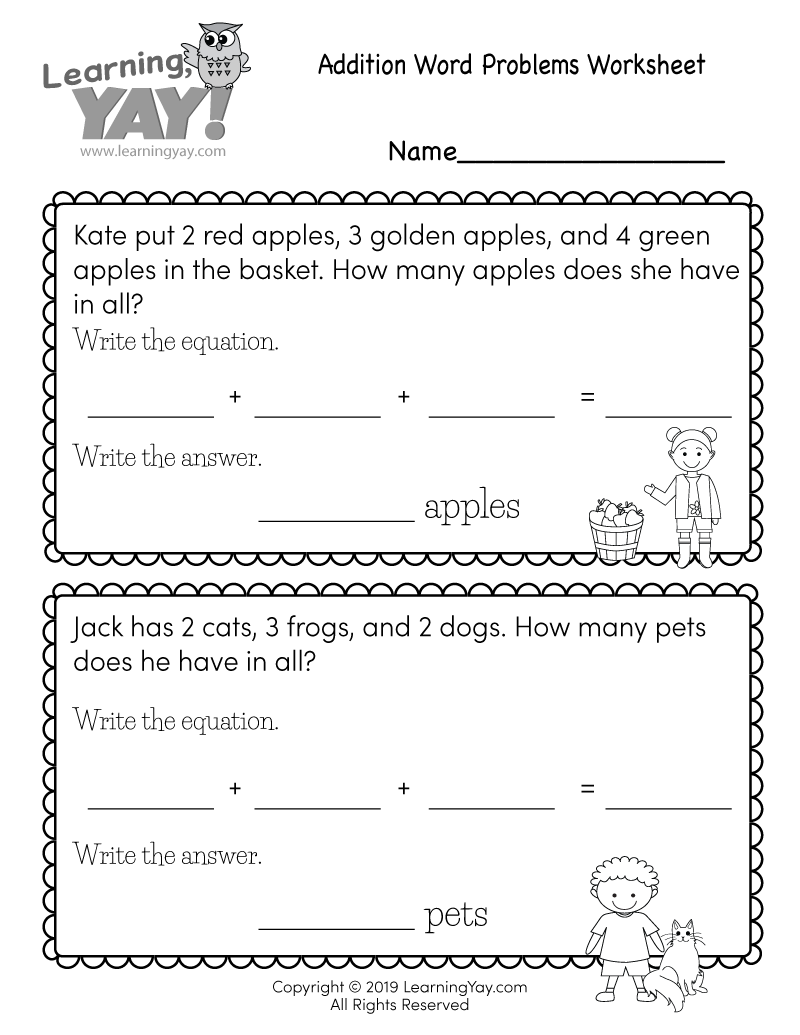# True or False Subtraction Worksheet

Students can improve their understanding of the equal sign by solving subtractions and then determining if the equations are true or false in this Common core aligned math worksheet for first grade.This free printable math worksheet is aligned to first grade Common Core standards. It is an excellent resource for teachers and parents who are teaching the following standard.
##### 1.OA.D.7 - Common Core ID

Understand the meaning of the equal sign, and determine if equations involving addition and subtraction are true or false. For example, which of the following equations are true and which are false? 6 = 6, 7 = 8 – 1, 5 + 2 = 2 + 5, 4 + 1 = 5 + 2.

Common Core » 1st Grade Math Standards » Operations & Algebraic Thinking » Work with addition and subtraction equations. » 1.OA.D.7

## There are multiple ways to get this worksheet.

### You might also like these math worksheets: